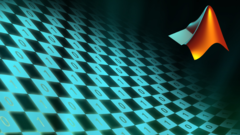## Introduction to Programming with MATLAB

 所在平台: Coursera 课程类别: 计算机科学Explore 1600+ online courses from top universities. Join Coursera today to learn data science, programming, business strategy, and more.#### 课程详情

MATLAB is a special-purpose language that is an excellent choice for writing moderate-size programs that solve problems involving the manipulation of numbers. The design of the language makes it possible to write a powerful program in a few lines. The problems may be relatively complex, while the MATLAB programs that solve them are relatively simple: relative, that is, to the equivalent program written in a general-purpose language, such as C++ or Java. As a result, MATLAB is being used in a wide variety of domains from the natural sciences through all disciplines of engineering to finance and beyond, and it is heavily used in industry. Hence, a solid background in MATLAB is an indispensable skill in today’s job market.

Nevertheless, this course is not a MATLAB tutorial. It is an introductory programming course that happens to use MATLAB to illustrate general concepts in computer science and programming.

Students who successfully complete this course will:
• become familiar with general concepts in computer science
• gain an understanding of the general concepts of programming
• obtain a solid foundation in the use of MATLAB
This is an introductory college-level course in computer science for engineering and science students. However, it is also suitable for high school students who are interested in programming. The material assumes no background in mathematics beyond the standard high school curriculum. Students taking the course will get a MATLAB license free of charge for a three-month period.

#### 课程大纲

The course is divided into the following 8 weekly modules:

Week 1: Introduction to MATLAB

Week 2: Matrices and Operators

Week 3: Functions

Week 4: Programmer’s Toolbox

Week 5: Selection

Week 6: Loops

Week 7: Data Types

Week 8: File Input/Output

#### 欢迎关注我们的公众号#### 课程简介

This course teaches computer programming to those with little to no previous experience. It uses the programming system and language called MATLAB to do so because it is easy to learn, versatile and very useful for engineers and other professionals.

matlab Matlab编程

#### 15人关注该课程